Next: 6.7 Numerical Evaluation of Up: 6 Quantum Diffusion II: Previous: 6.5 One-Phonon Quantum Diffusion

# 6.6 Experimental Results in Solid Xe

Muonium spin polarization spectra were measured in solid Xe by both TF and LF methods. The relaxation of the polarization in the LF case occurs as a result of the motion of the Mu atom among atoms of the host lattice, some of which are isotopes with nuclear moments. The nuclear hyperfine (nhf) interaction between the electron bound in the Mu atom and surrounding nuclear moments leads to an additional term in the Hamiltonian describing Mu spin dynamics: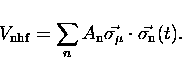(24)
This problem is made tractable by approximating this interaction with an effective time-dependent magnetic field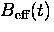which fluctuates both in direction and magnitude as a result of the motion of the Mu atom. The Fourier transform ofgives the spectral function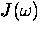of the fluctuating effective field. Transitions among the states of the Mu atom differing in energy by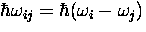are induced in proportion to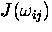, hence changing the muon spin state and irreversibly reducing the muon spin polarization. The resulting muon spin polarization function was calculated by Celio and Meier  and Celio and Yen [64,65].

For slowly diffusing muonium where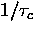is small compared to all transition frequencies except the smallest -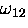- the relaxation function is well approximated by a simple exponential with a relaxation rate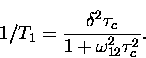(25)
The temperature dependence of this relaxation rate is shown in Fig. 6.27, demonstrating that, at least for temperatures from 40 to 120 K, the muonium hop rate increases with temperature.

In general the time evolution of the muon polarization is a complicated function of the inverse correlation time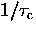, the exchange coupling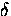and LF field B, which can be calculated numerically by the algorithm due to Celio. The muonium diffusion hop rateat each temperature is extracted from the LF data by simultaneously fitting the polarization functions measured in several different magnetic fields to the complete theoretical polarization function. The resultant temperature dependence of the Mu hop rate is shown in Fig. 6.28.

In order to elucidate the nature of the peculiar change in relaxation rate at temperatures above T=130 K, a TF experiment on a sample of solid 136Xe was also performed. The resulting relaxation rate 1/T2 is also shown in Fig. 6.27. Since 136Xe atoms do not have nuclear moments there should not be any contribution to spin relaxation due to dipolar broadening. Electric field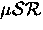experiments were unable to cause any change in the polarization function at any temperature, so we cannot be certain exactly what caused the addition relaxation, however it is probably of a chemical nature, and comes into effect at high temperatures where the muonium is most rapidly diffusing. We will therefore restrict ourselves to temperatures below 120 K where this anomalous contribution to the relaxation rate in longitudinal field is negligible.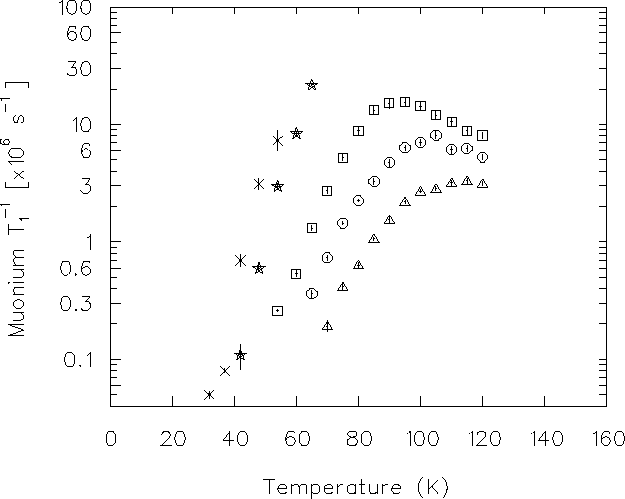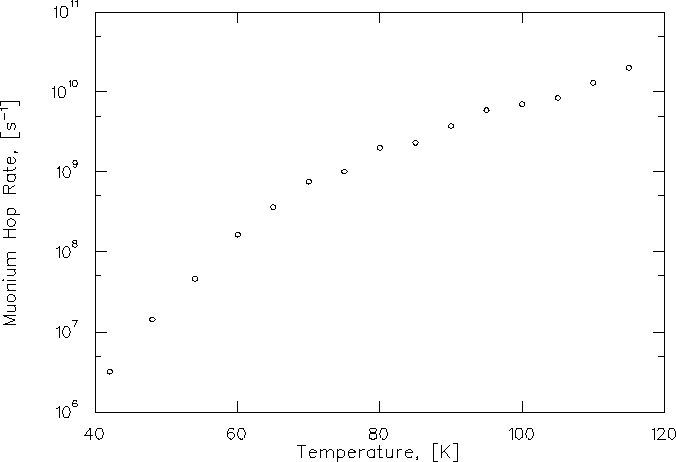The same results are also presented in the form of an Arrhenius plot in Fig. 6.30. The hop rate as a function of temperature can be fitted quite adequately to an Arrhenius function,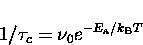(26)
representing a simple over-barrier classical hopping mechanism with hop attempt rateand activation energy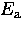.Such a fit yields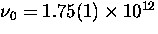s-1, about twice the Debye frequency, and a barrier height= 0.0482(2) eV. It is difficult to immediately rule out this mechanism on grounds of the barrier height, since it is not unreasonable. (We shall, however, discuss a calculation of the shape of the potential well of Mu in Xe in Section 6.8, which estimates the depth of the potential well to be about 1 eV.) On the other hand, the indicated attempt frequency is more than a factor of 10 smaller than what one expects. The attempt rate on the barrier in the case of over-barrier activation would be the zero-point frequency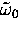of the particle in the well. Since the interaction between atoms of a van der Waals solid has the same origin as the barrier to diffusion of muonium atoms we can make a rough estimate ofby assuming that the interstitial occupies a site where it is subject to a potential similar to that which holds the lattice atoms at their equilibrium positions. Assuming a harmonic potential, we may scale the Debye frequency by the root of the ratio of the lattice atom's mass to the interstitial's mass: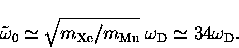(27)
Measurements of the vibrational frequencies of hydrogen and deuterium embedded in solid Xe agree quite well with this approximation. [58,60] Consequently, this result allows us to rule out classical over-barrier hopping being responsible for the observed hop rate since the expected frequency is about 17 times larger than the Arrhenius plot indicates.Next: 6.7 Numerical Evaluation of Up: 6 Quantum Diffusion II: Previous: 6.5 One-Phonon Quantum Diffusion